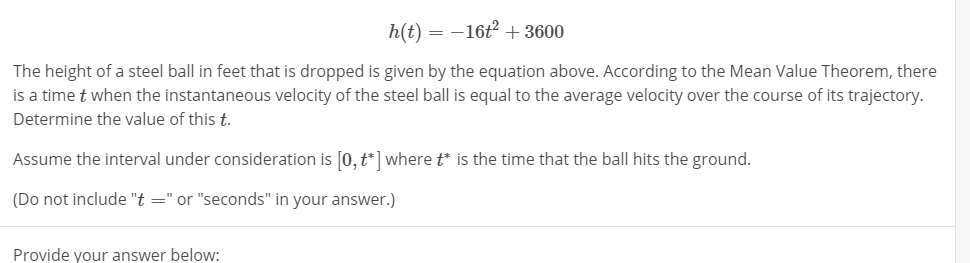# h(t)-16+3600The height of a steel ball in feet that is dropped is given by the equation above. According to the Mean Value Theorem, thereis a time t when the instantaneous velocity of the steel ball is equal to the average velocity over the course of its trajectoryDetermine the value of this t.Assume the interval under consideration is [o,t] wheretis the time that the ball hits the ground.(Do not include "t"or "seconds" in your answer.)Provide your answer below:

Question
664 viewshelp_outlineImage Transcriptioncloseh(t)-16+3600 The height of a steel ball in feet that is dropped is given by the equation above. According to the Mean Value Theorem, there is a time t when the instantaneous velocity of the steel ball is equal to the average velocity over the course of its trajectory Determine the value of this t. Assume the interval under consideration is [o,t] wheretis the time that the ball hits the ground. (Do not include "t"or "seconds" in your answer.) Provide your answer below: fullscreen
check_circle

Step 1

To determine the value of "t" as defined by the problem statement

Step 2

At time t* , h(t*)=0 (hits the ground). So, first determine t* (=15)

Step 3

### Want to see the full answer?

See Solution

#### Want to see this answer and more?

Solutions are written by subject experts who are available 24/7. Questions are typically answered within 1 hour.*

See Solution
*Response times may vary by subject and question.
Tagged in

### Derivative